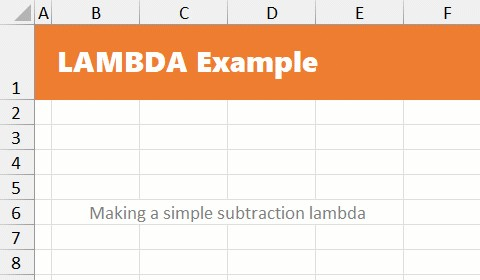# What is LAMBDA? 4 Practical examples to REALLY understand it

Ladies & gentlemen… Excel has a new function. LAMBDA()  is the new addition to Excel’s family of functions. Using LAMBDA, we can create custom & reusable functions in Excel.

• What is LAMBDA()
• How to create LAMBDAs in Excel?
• How to re-use LAMBDAs
• 4 Practical Examples of LAMBDA
• What are LAMBDA helper functions?
• Sharing LAMBDAs with others
• How LAMBDA compares with other custom function options in Excel?

### LAMBDA – Who can use it?

LAMBDA() and other lambda helper functions are available in below formats of Excel only.

• Excel 365
• Excel on the Web
• iOS & Android Excel

If you are using an older version of Excel (Excel 2019 / 2016…) then you can test and practice LAMBDA from Excel on the web. Just go to https://excel.new

## What is LAMBDA & How to create it?

LAMBDA (pronounced lam-duh) allows us to write our own functions and add them to the library.

For example, here is a very simple LAMBDA to subtract one value from another.

```				```
=LAMBDA(a, b, a-b)
```
```

To call this LAMBDA, you can use the below syntax.

```				```
=LAMBDA(a, b, a-b)(90, 12)

' The result will be 78
```
```

Here is a quick demo of our subtraction LAMBDA…## How to “install” & “reuse” LAMBDA?

The TRUE Power of LAMBDA is unleashed once you “install” or “setup” them. To install out subtract lambda,

1. Go to Formulas ribbon and click on “Define Name”.
2. Type a name for the lambda, such as subtract
3. In the “Refers to:” area of the name, type your lambda. For ex:
1. =LAMBDA(a, b, a-b)
4. Click OK.
5. That is all. Our SUBTRACT LAMBDA is setup.

To call the lambda, just go to any cell and type the =subtract formula and provide parameters a & b as you normally would.

How awesome is that.Here is a quick demo of the LAMBDA setup process.## Practical Examples of LAMBDA

Let’s be honest. No one needs the SUBTRACT function. We can already do that with the – (minus) operator. So in this part of the article, let me present some practical LAMBDAs.

Calculating weighted averages is a pain in Excel. There is no built-in function to do the task. Well, we can make our own WAVERAGE() function, thanks to LAMBDA().

Define WAVERAGE name as

=LAMBDA(values, weights, SUMPRODUCT(values, weights)/ SUM(weights))

Here is how you can use it to correctly calculate weighted average of salaries.Let’s say you have a list of values and just want to get the “topn” values in descending order. We can set up a LAMBDA to do that magic.

Define TOPN name as

=LAMBDA(values, n, LARGE(values, SEQUENCE(n)))

We can call this LAMBDA like:

=TOPN(a1:a10, 3) to see top 3 values in the range A1:A10.

Excel has the excellent TODAY() function to tell me what is the current date. But there is no TOMORROW()?

Let’s fix that with LAMBDA.

Define the name tomorrow as,

=LAMBDA(TODAY()+1)

And, you can call it by writing =TOMORROW() in any cell. Works like magic…

You can write complex Date logic to figure that out. But who wants to write the same formulas every time. This is the job for LAMBDA.

Define FirstMonday as,

=LAMBDA(anydate,
LET(som, DATE(YEAR(anydate),MONTH(anydate),1), CHOOSE(WEEKDAY(som),1,0,6,5,4,3,2)+som))

And to get the first Monday of any month, you can use =FIRSTMONDAY(any date)

For example, =FIRSTMONDAY(“1-jan-2022”) will be 3-Jan-2022 (which is the first monday).

## What are LAMBDA “helper” functions…?

Apart from LAMBDA, Microsoft also introduced a bunch of helper functions so we can truly build any kind of LAMBDAs in Excel.

These are,

• MAP – to map (or apply) a LAMBDA to a range of values and return a range of outputs.
• REDUCE – to take a bunch of values (or lambda outputs) and reduce them to a single value
• SCAN – Returns intermediate results of applying a lambda on an array – one step at a time.
• MAKEARRAY – gives you an array of specific size (rows by columns) by applying the lambdas
• BYCOL – applies a lambda function to a range, one column at a time and returns a row of outputs.
• BYROW – revers of BYCOL…

## How to “share” LAMBDAs with others?

By default, any LAMBDA you create is only available in the workbook. To share LAMBDAs across files or with other users, you can try:

• Simpler option: Email or share the files with others. When they open the file, they can see & use the LAMBDAs in that file or copy paste the names to their own files.
• Better option: You can use the newly added “Advanced Formula Editor” to share and centrally publish lambdas (with Github). This is for advanced users only. You can get the add-on here.

## How does LAMBDA compare with UDFs?

Seasoned users of Excel know that we already have the ability to create and share our own functions. It is called user defined functions (or UDFs).

Here are some points on how UDFs compare with LAMBDA.

• UDFs rely on VBA (or add-ins). If you disable the add-in or don’t load the VBA code, then the UDF won’t work.
• LAMBDAs are part of core Excel, so they will run without any permission or loading.
• UDFs are easy to share, but LAMBDAs have no such option yet.
• UDFs work in all desktop versions of Excel. LAMBDA is on available in Excel 365 but they also work on Excel on Web & on apps.
• UDFs let you access Visual Basic object model (restricted) and do things on your spreadsheet that are not possible with formulas (for ex: extract comment in a cell or find the color of a cell).